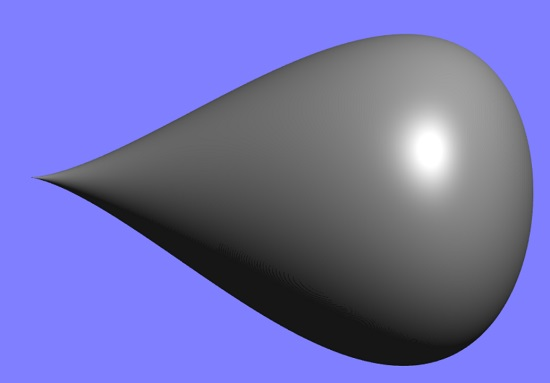# Piriform CurveThe piriform curve (also called the peg-top curve or pear-shaped quartic) is a teardrop-shaped family of curves given by the Cartesian equation

• a4 y2 = b2 x3 (2a – x)

where the curve’s midline lies on the x-axis and

• y = (o, ±b) for x = a.

The area of a pirifrom is the same as the area of an ellipse with semiaxes a and b .

It can also be described by the algebraic equation

• x4 – 2ab2 x3 + a4 y2 = 0
• x = a (1 + sint) and y = b cost (1 + sint).

This famous curve, which is symmetric about the x-axis, is named after the Latin pirum “pear” and appears in many places in nature including some birds eggs, seeds, and part of the human nasal passage.

The piriform was first studied by French mathematician Gaston Albert Gohierre de Longchamps in 1866 .

## Construction of the Piriform Curve

The piriform curve can be constructed as follows: Given a point A on the circumference of a circle and a line L1 perpendicular to the diameter through point A:

• Draw a line through point A that crosses L1 in point B.
Draw a second line, L2, perpendicular to L1, intersecting the circle at point C.
• Draw a third line L3, perpendicular to L2 through point C and intersecting line L in point P.

The piriform curve is the locus of P for all possible lines Ln .

## Piriform Curve Derivatives

for the parametric representation x = a (1 + sint) and y = b cost (1 + sint), the derivatives are:## References

Top image: Mike Williams, CC BY-SA 3.0, via Wikimedia Commons
 Unger, J. How Wasanka Did Integration: The Case of the Japanese Wedge. Sangaku Journal of Mathematics (SJM). Volume 5 (2021), pp. 43–55.
 Cole, D. Playing with Dynamic Geometry.

CITE THIS AS:
Stephanie Glen. "Piriform Curve" From StatisticsHowTo.com: Elementary Statistics for the rest of us! https://www.statisticshowto.com/piriform-curve/